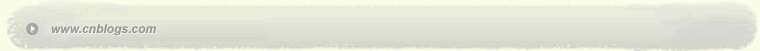Impossible is nothing 爱过知情重醉过知酒浓 　　花开花谢终是空 　　缘份不停留像春风来又走 　　女人如花花似梦

 < 2007年5月 >
293012345
6789101112
13141516171819
20212223242526
272829303112
3456789

• 随笔 - 8
• 文章 - 91
• 评论 - 16
• 引用 - 0

# 相册

•

### 评论排行榜

区域填充即给出一个区域的边界，要求对边界范围内的所有象素单元赋予指定的颜色代码。区域填充中最常用的是多边形填色，本节中我们就以此为例讨论区域填充算法。

1、将多边形画在纸上。

2、用 一根水平扫描线自左而右通过多边形而与多边形之边界相交。扫描线与边界相交奇次数后进入该多边形，相交偶次数后走出该多边形。图2.3.1示出这类情况： 扫描线与多边形相交四点。相交a点之后入多边形；交b点（第2交点）之后出多边形；交c点（第3交点）之后又入多边形；交d点（第4交点）之后又出多边 形。图2.3.1                                                                            图2.3.2

1、扫描线填色（Scan-Line Filling）算法。这类算法建立在多边形边边界的矢量形式数据之上，可用于程序填色，也可用交互填色。

2、种子填色（Seed Filling）算法。这类算法建立在多边形边边界的图象形式数据之上，并还需提供多边形界内一点的坐标。所以，它一般只能用于人机交互填色，而难以用于程序填色。

区域填充即给出一个区域的边界，要求对边界范围内的所有象素单元赋予指定的颜色代码。区域填充可以分两步走，第一步先确定需要填充那些象素，第二步确定用 什么颜色来填充。区域填充中最常用的是多边形填色。

1>.扫描线填色算法

1. 左、右顶点处理 当以1, 2, 3的次序画多边形外框时，多边形的左顶点和右顶点如图2.3.3 (a)、(b)所示的顶点2。它们具有性质：

(a)左顶点 (b)右顶点2. 水平边处理 水平边（y1=y2）与水平扫描线重合法求交点。因此，将水平边画出后删去，不参加求交及求交以后的操作。

3. 扫描线与边的求交点方法采用递归算法 边（x1, y1）（x2, y2）与扫描线i+1的交点为：（当交点不为x1, y1时）

4. 减少求交计算，采用活性边表 对于一根扫描线而言，与之相交的边只占多边形全部边的一部分。因此，在基本算法思想中，每根扫描线与多边形所有边求交的操作是一种浪费，需要加以改善。活 性边表（Active List of Side）的采用将多边形的边分成两个子集：与当前扫描线相交的边的集合，以及与当前的扫描线不相交的边的集合。后者不必予以求交，这样就提高了算法的效 率。

(a) (b)在scan1的情况1） 将经过左、右顶点处理及剔除水平边后的多边形之各边按照max y值排序，存入一个线性表中。表中每一个元素代表一根边。第一个元素是max y值最大的边，最后一个元素是max y值最小的边。图2.3.4 (a)中的多边形所形成的线性表如（b）所示。其中F点和B点的y值相等，且为全部多边形的max y的最大值。因此FG, FE, AB, BC等四边排在表之首。而C点的y值>E点的y值，所以CH排在DE前面，余类推。在max y值相等的边之间，按任意次序排列。

2） 在上述线性表上加入两个指针first和last，即形成活性边表。这两个指针之间是与当前扫描线相交的边的集合和已经处理完（即扫描完）的边的集合。这 两者的区分方法是在处理完的边上加上记号：? y=0。在last指针以后的是尚未与当前扫描线相交的，在first指针以前的是已经处理完了的边。对于图2.3.4 (a)中扫描线scan1的情况下，图2.3.4 (b)中列出first, last的位置。如果扫描线由上而下移到了scan2的位置，则活性边表的first应指向AB，last应指向CH。每根扫描线只须与位于first, last之间的，而且? y? 0的边求交即可。这就缩小了求交的范围。

3）活性边表中每个元素的内容包括：

·边的max y值，记为y_top；

·与当前扫描线相交点的x坐标值，记为x_int；

·边的y方向当前总长。初始值为y2-y1。记为? y；

·边的斜率倒数：，记为x_change_per_scan。

4）活性边在每根扫描线扫描之后刷新。刷新的内容有2项：

·调整first和last指针字间的参加求交的边元素之值：? y=? y-1; x_int = x_int - x_change_per_scan;

·调整first和last指针，以便让新边进入激活范围，处理完的边退出激活范围：图2.3.5 fill_area的程序结构

typedef struct {

int y_top;

float x_int;

int delta_y;

floaat x_change_per_scan;

} EACH_ENTRY;

EACH_ENTRY SIDES[MAX_POINT];

int x[MAX_POINT], y[MAX_POINT];

int side_count, first_s, last_s, scan, bottomscan, x_int_count, r;

fill_area(count, x, y)

int count, x[ ], y[ ];

{

sort_on_bigger_y(count);

first_s=1;

last_s=1;

for (scan=sides.y_top; scan>bottomscan ?; scan - -)

{

up date_first_and_last(count, scan);

process_x_intersections(scan, first_s, last_s);

draw_lines (scan, x_int_count, first_s);

update-_sides_list ( );

}

}

void put_in_sides_list(entry, x1, y1, x2, y2, next_y);

int entry, x1, y1, x2, y2, next_y;

{

int maxy;

float x2_temp, x_change_temp;

x_change_temp = (float) (x2-x1) / (float) (y2-y1);

x2_temp =x2; /*以下为退缩一点操作. */

if ((y2>y1) && (y2<next_y)) {

y2 - - ;

x2_temp - = x_change_temp;

}

else {

if ((y2<y1) && (y2 >next_y)) {

y2++;

x2_temp+=x_change_temp;

}

}

/* 以下为插入活性表操作. */

maxy = (y1 > y2)? y1: y2;

while (( entry >1) && (maxy > sides [entry -1]. y_top))

{

sides[entry]=sides [entry ?];

entry - -;

}

sides[entry]. y_top=maxy;

sides[entry]. delta_y =abs(y2-y1)+1;

if (y1>y2)

sides[entry]. x_int =x1;

else{

sides[entry].x_int=x2_temp;

sides[entry]. x_change_per_scan=x_change_temp;

}

void sort_on_bigger_y(n)

int n;

{

int k, x1, y1;

side_count=0;

y1=y[n];

x1=x[n];

bottomscan=y[n];

for (k=1; k<n+1; k++)

{

if (y1 ! =y[k]) {

side_count ++;

put_in_sides_list(side_count, x1, y1, x[k], y[k]);

}

else {

move ((short)x1, (short)y1);

line((short)x[k], (short)y1, status);

}

if (y[k] <bottomscan) bottomscan=y[k];

y1=y[k]; x1=x[k];

}

}

void update_first_and_last(count, scan)

int count, scan;

{

while((sides[last_s+1]. y_top>=scan) && (last_s <count)) last_s ++;

while(sides[first_s]. delta_y = = 0) first_s ++;

}

void swap(x, y)

EACH_ENTRY x, y;

{

int i_temp;

float f_temp;

i_temp=x.y_top; x.y_top=y.y_top; y.y_top=i_temp;

f_temp=x.x_int; x.x_int=y.x_int; y.x_int=f_temp;

i_temp=x.delta_y; x.delta=y.delta_y; y.delta_y=i_temp;

f_temp=x.x_change_per_scan; x. x_change_per_scan=y. x_change_per_scan; y.x.

change_per_scan=f_temp;

}

void sort_on_x(entry, first_s)

int entry, first_s;

{

while((entry > first_s) && (sides[entry]. x_int < sides[entry-1]. x_int))

{

swap (sides[entry], sides[entry-1]);

entry - -;

}

}

void process_x_intersections(scan, first_s, last_s)

int scan, first_s, last_s;

{

int k;

x_int_cout=0;

for(k=first_s; k<last_s+1; k++)

{

if(sides[k]. delta_y >0) {

x_int_count ++;

sort_on_x(k, first_s);

}

}

}

void draw_lines(scan, x_int_count, index)

int scan, x_int_count, index;

{

int k, x, x1, x2;

for (k=1; k< (int) (x_int_count/2+1.5); k++)

{

while(sides[index]. delta_y = = 0) index ++;

x1=(int)(sides[index]. x_int +0.5);

index ++;

while(sides[index].delta_y = = 0) index ++;

x2 = (int) (sides [index]. x_int +0.5);

move((short)x1, (short)scan);

line((short)x2, (short)scan, status);

index ++;

}

}

void update_sides_list( )

{

int k;

for (k=first_s; k<last_s +1; k++)

{

if(sides[k].delta_y >0)

{

sides[k].delta_y - -;

sides[k]. x_int - = sides[k]. x_change_per_scan;

}

}

}

程序2.3.1 扫描线填色程序

1、sort_on_bigger_y子程序的主要功能是按照输入的多边形，建立起活性边表。操作步骤是：对每条边加以判断：如非水平边则调用put_in_side_list子程序放入活性边来；如是水平边则直接画出。

2、put_in_sides_list子程序的主要功能是将一条边存入活性边表之内。操作步骤是：对该边判别是否左顶点或右顶点，如果将入边之终点删去，按照y_top的大小在活性边表中找到该点的合适位置，在该边的位置中填入数据。

3、update_first_and_last子程序的主要功能是刷新活性边表的first和last两根指针的所指位置，以保证指针指出激活边的范围。

4、 process_x_intersections子程序的主要功能是对活性边表中的激活边（即位于first和last之间的，并且? y? 0的边）按照x_int的大小排序。操作步骤是：从first到last，对每一根? y? 0的边，调用sort_on_x子程序排入活性边表中合适位置。

5、 sort_on_x子程序主要功能是将一条边side[entry]，在活性边表的first到entry之间按x_int的大小插入合适位置。操作步骤 是：检查位于entry的边的x_int是否小于位置entry-1的边的x_int，如是，调用swap子程序交换两条边的彼此位置。

6、swap子程序的主要功能是交换活性边表中两条相邻位置边的彼此位置。

7、draw_lines子程序的主要功能是在一条扫描线位于多边形内的部分，填上指定的色彩。操作步骤是：在活性边表的激活边范围内，依次取出Δy¹ 0两边的x_int，作为两个端点（x1, scan），(x2, scan)，画一条水平线。

8、update_sides_list子程序的主要功能是刷新活性边表内激活边的值：Δy=Dy-1

x_int=x_int_x_chang_per_scan；2>种子填色算法

void seed_filling (x, y, fill_color, boundary_color)

int x, y, fill_color, boundary_color;

{

int c;

c=inquire_color(x, y);

if((c< > boundary_color) && (c< > fill_color))

{

set_pixel(x, y, fill_color);

seed_filling(x+1, y, fill_color, boundary_color);

seed_filling(x-1, y, fill_color, boundary_color);

seed_filling(x, y+1, fill_color, boundary_color);

seed_filling(x, y-1, fill_color, boundary_color);

}

}

程序2.3.2 四邻法种子填色程序图2.3.6 四邻法和八邻法种子

1. 四邻法

2. 四邻法不能填满此多边形

3. 八邻法

4. 八邻法会涂出此多边形

posted on 2006-02-24 22:38 笑笑生 阅读(3319) 评论(1)  编辑 收藏 引用• # re: 区域填充算法  lishali Posted @ 2007-05-24 20:13
顶顶顶顶顶顶顶顶
顶顶顶顶顶顶顶顶顶顶顶顶顶顶顶顶顶顶顶顶

顶顶顶顶
顶顶顶顶
顶顶顶顶顶顶顶顶顶顶顶顶顶顶顶顶顶顶顶顶顶顶顶顶
顶顶顶顶  回复  更多评论Copyright © 笑笑生 Powered by: 博客园 模板提供：沪江博客